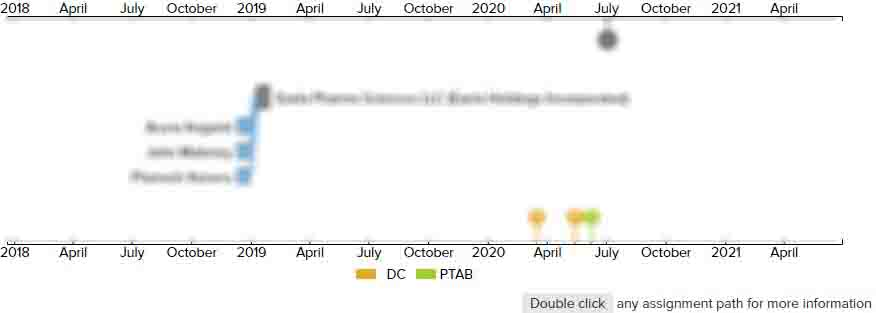# RECHARGEABLE BATTERY WITH NONAQUEOUS ELECTROLYTE, ITS NEGATIVE ELECTRODE, AND ITS MATERIAL

• US 20090181304A1
• Filed: 05/15/2006
• Published: 07/16/2009
• Est. Priority Date: 05/16/2005
• Status: Active Grant
##### First Claim
Patent Images

1. A negative electrode material for a nonaqueous electrolyte rechargeable battery in the form of particles comprising, at least on the surface thereof, a compound of the phase in which an element Z is present in Si in a non-equilibrium state, whereinthe compound contains Si, the element Z and another element M in the composition expressed by a general formula SiZxMy (where Z, x, M and y follow the conditions described below),the element Z is at least one of C and N,x is the value determined in such a manner that, when the concentration of Z in a compound SiaZp (where each of a and p is an integer) having a composition closest to that of Si and present in an equilibrium state is expressed by a formula p/(a+p), the Z concentration ratio Q(Z) expressed by the following formula is in the range of 0.10 to 0.95:

• Q(Z)=[x/(1+x)]/[p/(a+p)]the element M is one element or two or more elements selected from elements other than Si and the element Z, andy is a number within the range of 0 to 0.5.

• ##### 3 Assignments
Timeline View
Assignment View×
×